# RD Sharma Solutions for Class 11 Chapter 2 - Relations Exercise 2.2

Meta title: RD Sharma Solutions for Class 11 Chapter 2 – Relations Exercise 2.2 avail free pdf.

Meta disc: RD Sharma Solutions for Class 11 Chapter 2 – Relations Exercise 2.2 is given here, which provides solutions to questions related to some results on the cartesian product of sets.

In this section, we intend to study some results on the cartesian product of sets, which are given as theorems. For any doubts on how to solve the problems, students can refer to RD Sharma Class 11 Solutions which are available for students anytime. Exercise wise solutions are solved and prepared by our experts in a step by step manner which can be easily understandable for any student. The pdf of RD Sharma Class 11 Maths can be easily downloaded from the links provided below and students can start practising offline.

## Download the Pdf of RD Sharma Solutions for Class 11 Maths Exercise 2.2 Chapter 2 – Relations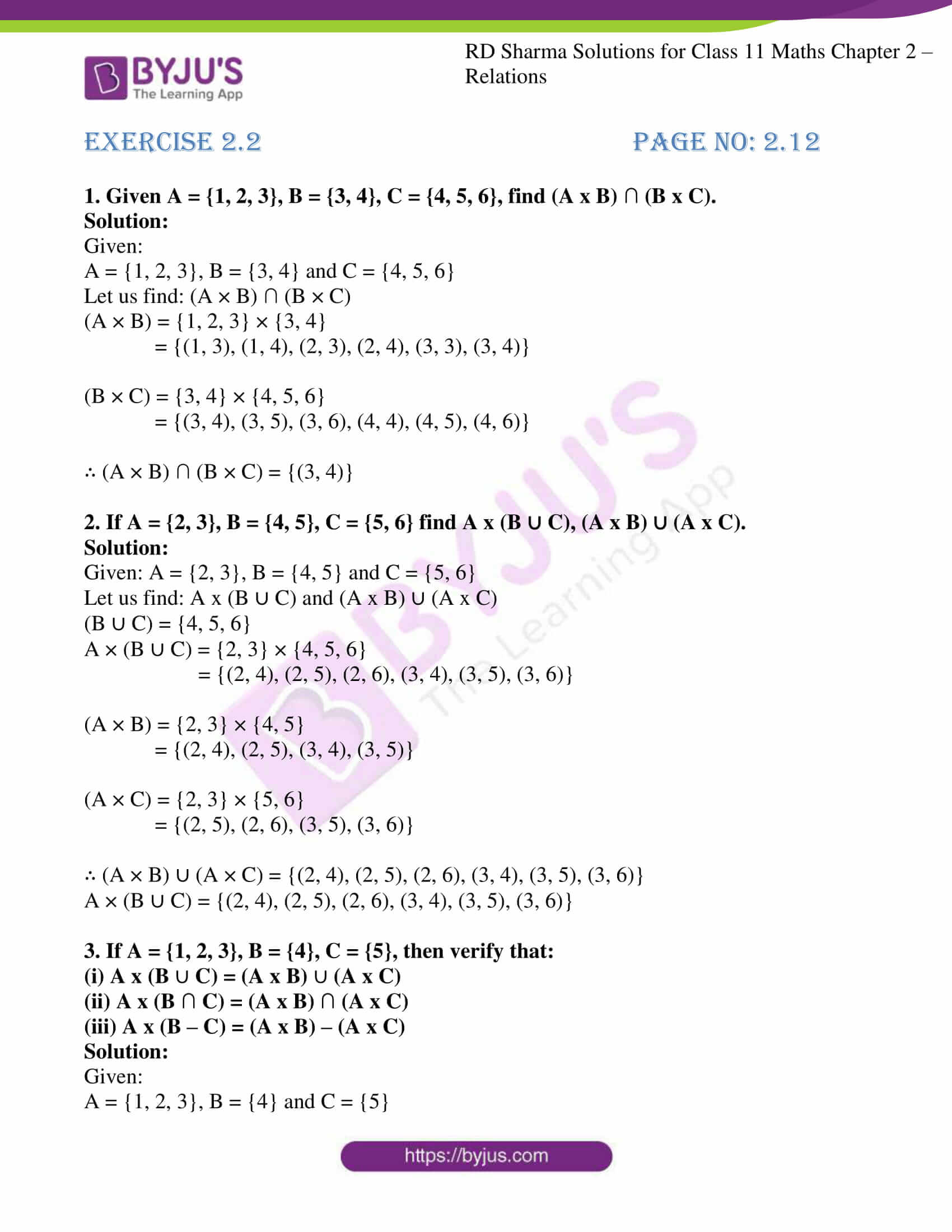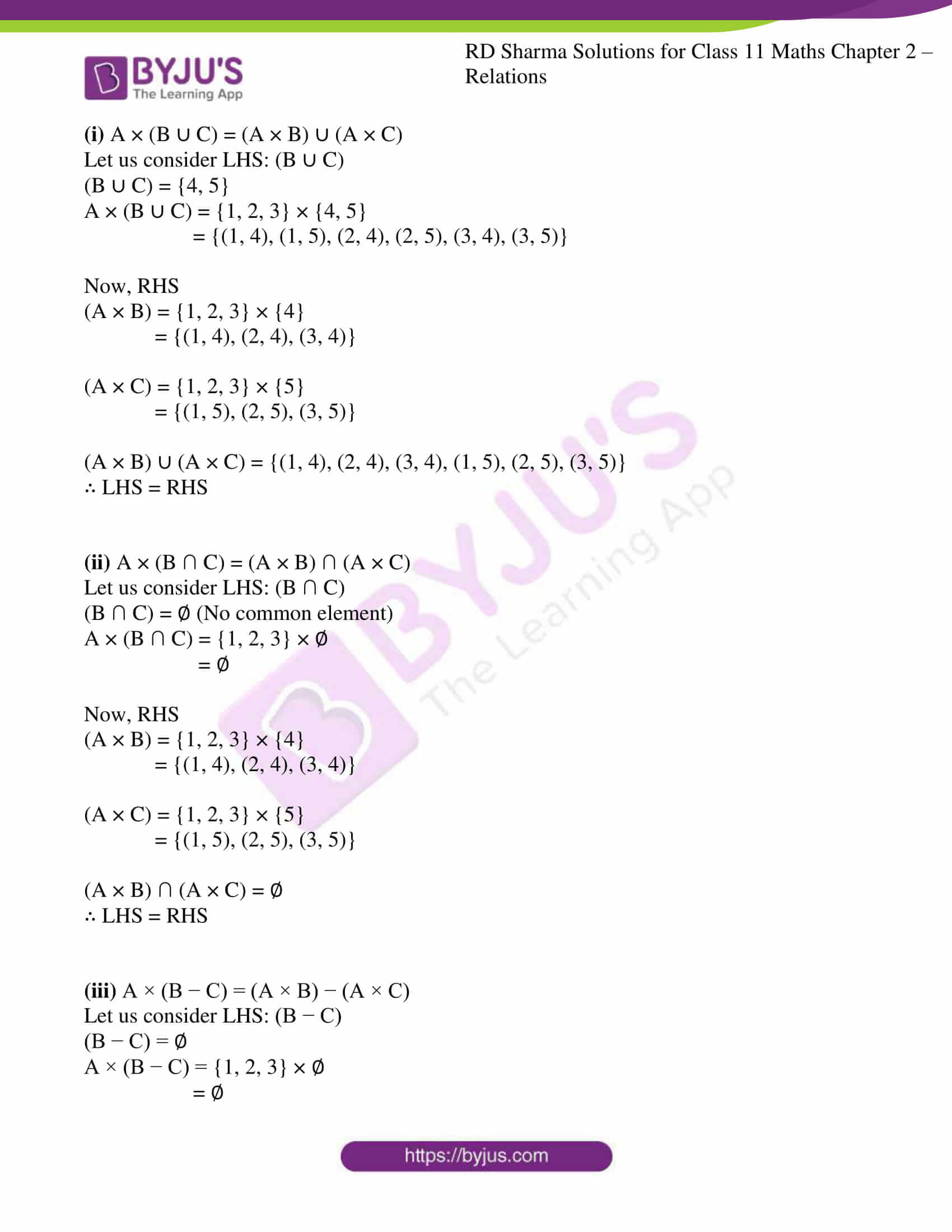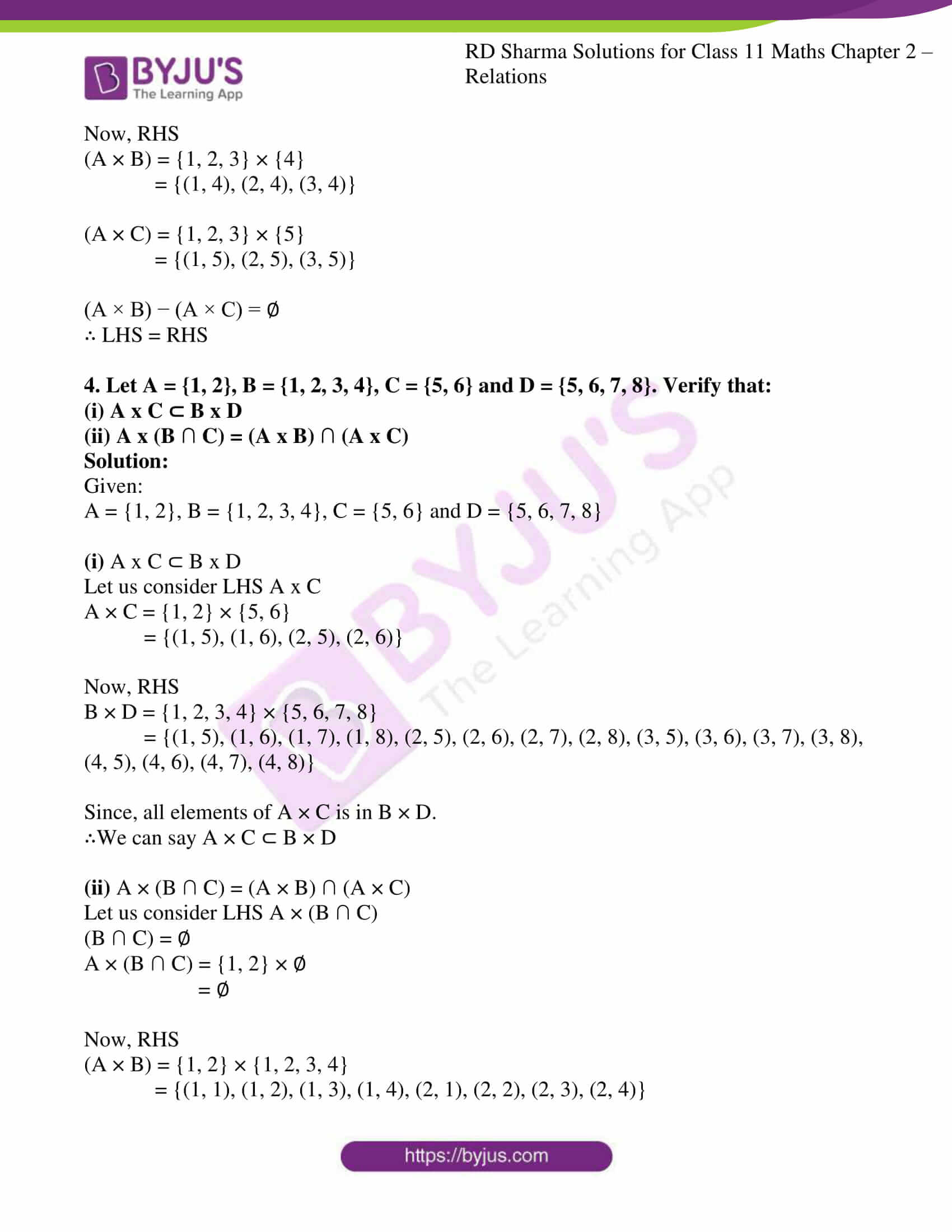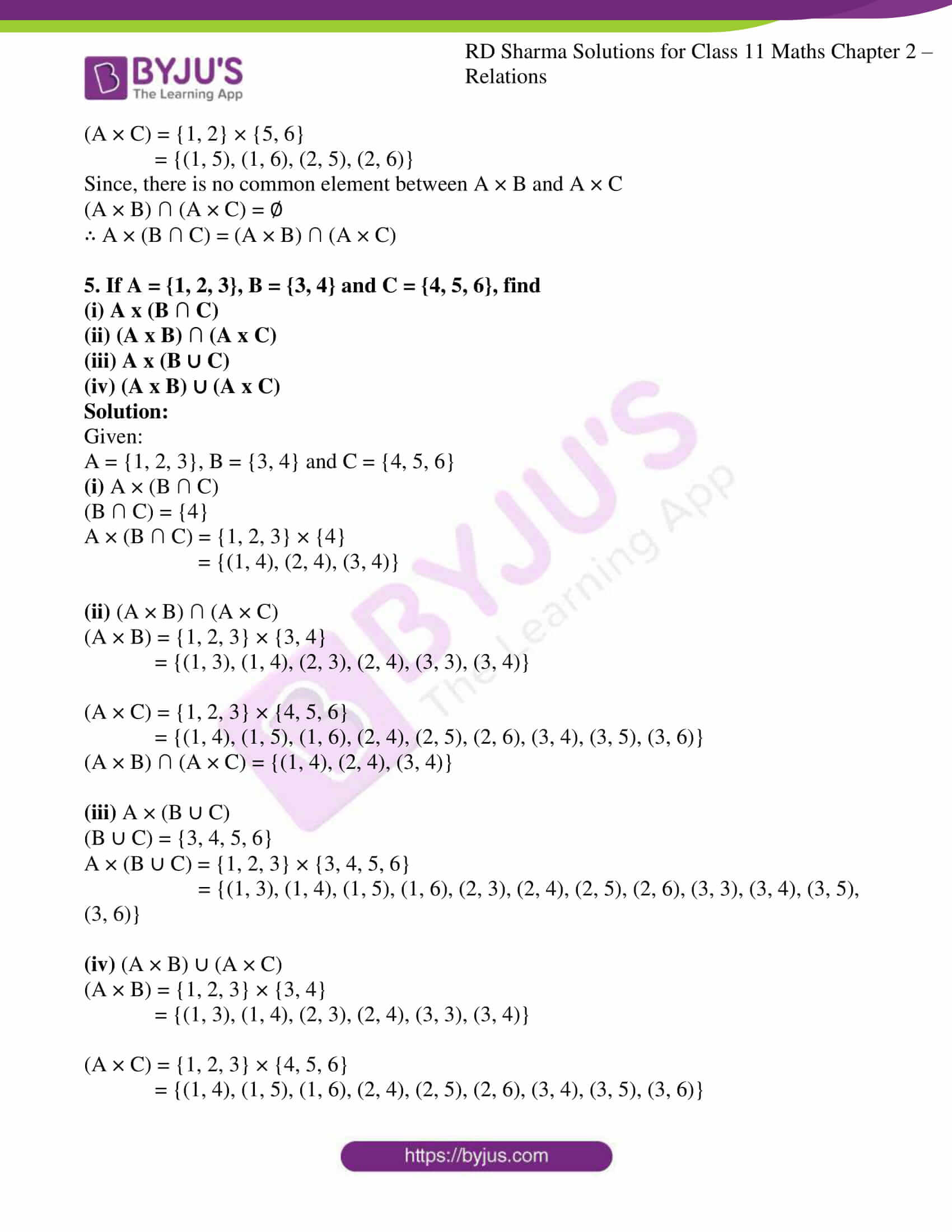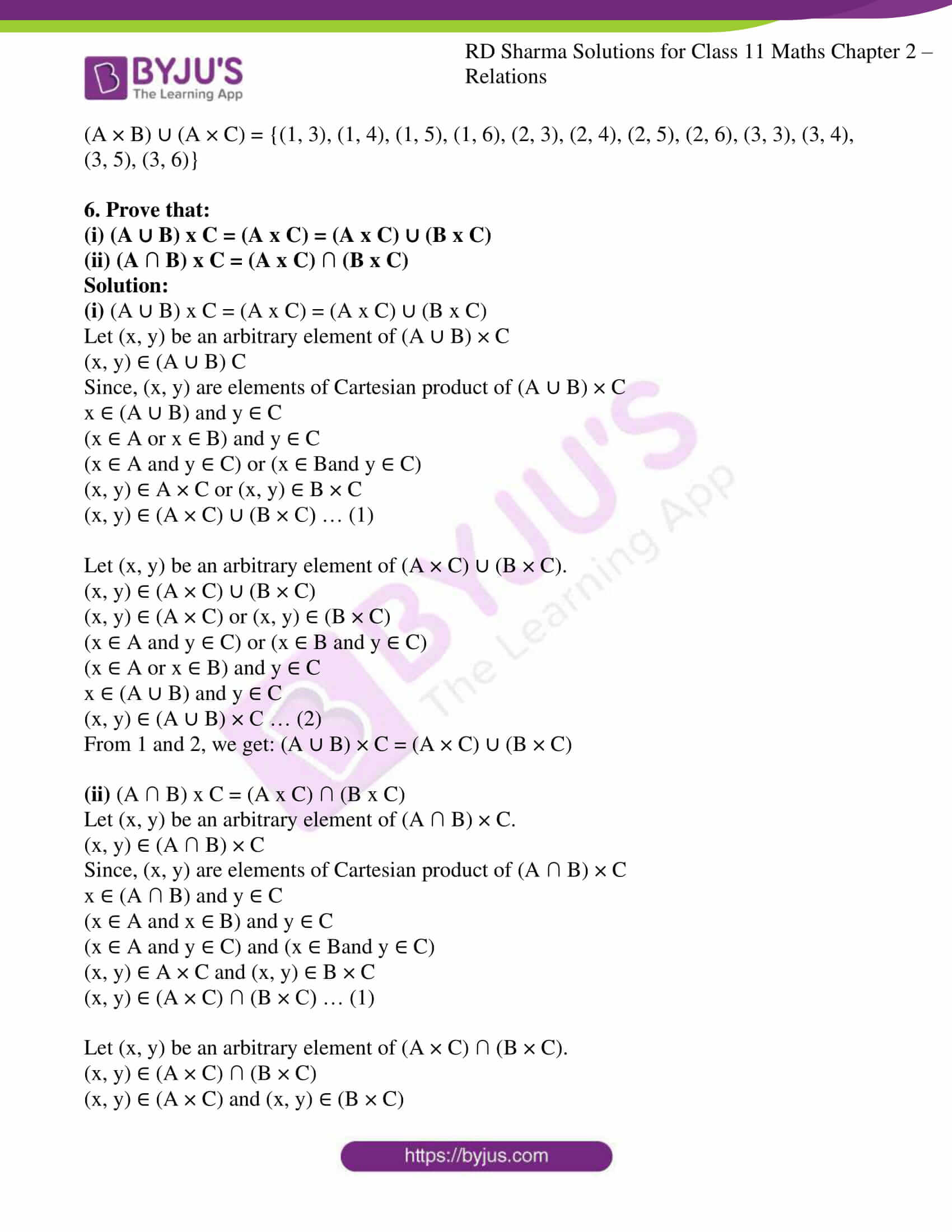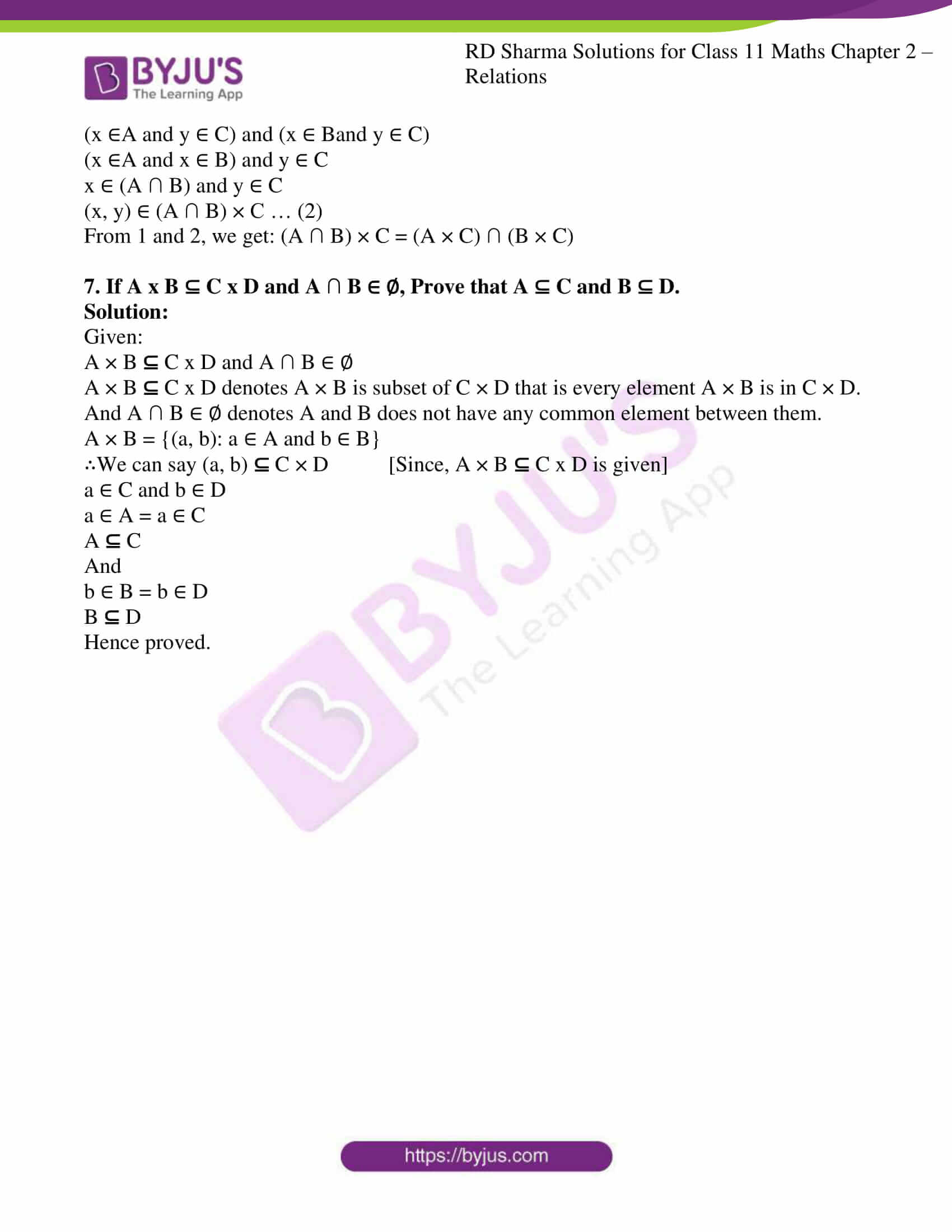### Access answers to RD Sharma Solutions for Class 11 Maths Exercise 2.2 Chapter 2 – Relations

1. Given A = {1, 2, 3}, B = {3, 4}, C = {4, 5, 6}, find (A x B) ∩ (B x C).

Solution:

Given:

A = {1, 2, 3}, B = {3, 4} and C = {4, 5, 6}

Let us find: (A × B) ∩ (B × C)

(A × B) = {1, 2, 3} × {3, 4}

= {(1, 3), (1, 4), (2, 3), (2, 4), (3, 3), (3, 4)}

(B × C) = {3, 4} × {4, 5, 6}

= {(3, 4), (3, 5), (3, 6), (4, 4), (4, 5), (4, 6)}

∴ (A × B) ∩ (B × C) = {(3, 4)}

2. If A = {2, 3}, B = {4, 5}, C = {5, 6} find A x (B ∪ C), (A x B) ∪ (A x C).

Solution:

Given: A = {2, 3}, B = {4, 5} and C = {5, 6}

Let us find: A x (B ∪ C) and (A x B) ∪ (A x C)

(B ∪ C) = {4, 5, 6}

A × (B ∪ C) = {2, 3} × {4, 5, 6}

= {(2, 4), (2, 5), (2, 6), (3, 4), (3, 5), (3, 6)}

(A × B) = {2, 3} × {4, 5}

= {(2, 4), (2, 5), (3, 4), (3, 5)}

(A × C) = {2, 3} × {5, 6}

= {(2, 5), (2, 6), (3, 5), (3, 6)}

∴ (A × B) ∪ (A × C) = {(2, 4), (2, 5), (2, 6), (3, 4), (3, 5), (3, 6)}

A × (B ∪ C) = {(2, 4), (2, 5), (2, 6), (3, 4), (3, 5), (3, 6)}

3. If A = {1, 2, 3}, B = {4}, C = {5}, then verify that:
(i) A x (B
C) = (A x B)  (A x C)
(ii) A x (B ∩ C) = (A x B) ∩ (A x C)
(iii) A x (B – C) = (A x B) – (A x C)

Solution:

Given:

A = {1, 2, 3}, B = {4} and C = {5}

(i) A × (B ∪ C) = (A × B) ∪ (A × C)

Let us consider LHS: (B ∪ C)

(B ∪ C) = {4, 5}

A × (B ∪ C) = {1, 2, 3} × {4, 5}

= {(1, 4), (1, 5), (2, 4), (2, 5), (3, 4), (3, 5)}

Now, RHS

(A × B) = {1, 2, 3} × {4}

= {(1, 4), (2, 4), (3, 4)}

(A × C) = {1, 2, 3} × {5}

= {(1, 5), (2, 5), (3, 5)}

(A × B) ∪ (A × C) = {(1, 4), (2, 4), (3, 4), (1, 5), (2, 5), (3, 5)}

∴ LHS = RHS

(ii) A × (B ∩ C) = (A × B) ∩ (A × C)

Let us consider LHS: (B ∩ C)

(B ∩ C) = ∅ (No common element)

A × (B ∩ C) = {1, 2, 3} × ∅

= ∅

Now, RHS

(A × B) = {1, 2, 3} × {4}

= {(1, 4), (2, 4), (3, 4)}

(A × C) = {1, 2, 3} × {5}

= {(1, 5), (2, 5), (3, 5)}

(A × B) ∩ (A × C) = ∅

∴ LHS = RHS

(iii) A × (B − C) = (A × B) − (A × C)

Let us consider LHS: (B − C)

(B − C) = ∅

A × (B − C) = {1, 2, 3} × ∅

= ∅

Now, RHS

(A × B) = {1, 2, 3} × {4}

= {(1, 4), (2, 4), (3, 4)}

(A × C) = {1, 2, 3} × {5}

= {(1, 5), (2, 5), (3, 5)}

(A × B) − (A × C) = ∅

∴ LHS = RHS

4. Let A = {1, 2}, B = {1, 2, 3, 4}, C = {5, 6} and D = {5, 6, 7, 8}. Verify that:
(i) A x C ⊂ B x D
(ii) A x (B ∩ C) = (A x B) ∩ (A x C)

Solution:

Given:

A = {1, 2}, B = {1, 2, 3, 4}, C = {5, 6} and D = {5, 6, 7, 8}

(i) A x C ⊂ B x D

Let us consider LHS A x C

A × C = {1, 2} × {5, 6}

= {(1, 5), (1, 6), (2, 5), (2, 6)}

Now, RHS

B × D = {1, 2, 3, 4} × {5, 6, 7, 8}

= {(1, 5), (1, 6), (1, 7), (1, 8), (2, 5), (2, 6), (2, 7), (2, 8), (3, 5), (3, 6), (3, 7), (3, 8), (4, 5), (4, 6), (4, 7), (4, 8)}

Since, all elements of A × C is in B × D.

∴We can say A × C ⊂ B × D

(ii) A × (B ∩ C) = (A × B) ∩ (A × C)

Let us consider LHS A × (B ∩ C)

(B ∩ C) = ∅

A × (B ∩ C) = {1, 2} × ∅

= ∅

Now, RHS

(A × B) = {1, 2} × {1, 2, 3, 4}

= {(1, 1), (1, 2), (1, 3), (1, 4), (2, 1), (2, 2), (2, 3), (2, 4)}

(A × C) = {1, 2} × {5, 6}

= {(1, 5), (1, 6), (2, 5), (2, 6)}

Since, there is no common element between A × B and A × C

(A × B) ∩ (A × C) = ∅

∴ A × (B ∩ C) = (A × B) ∩ (A × C)

5. If A = {1, 2, 3}, B = {3, 4} and C = {4, 5, 6}, find
(i) A x (B ∩ C)
(ii) (A x B) ∩ (A x C)
(iii) A x (B ∪ C)
(iv) (A x B) ∪ (A x C)

Solution:

Given:

A = {1, 2, 3}, B = {3, 4} and C = {4, 5, 6}

(i) A × (B ∩ C)

(B ∩ C) = {4}

A × (B ∩ C) = {1, 2, 3} × {4}

= {(1, 4), (2, 4), (3, 4)}

(ii) (A × B) ∩ (A × C)

(A × B) = {1, 2, 3} × {3, 4}

= {(1, 3), (1, 4), (2, 3), (2, 4), (3, 3), (3, 4)}

(A × C) = {1, 2, 3} × {4, 5, 6}

= {(1, 4), (1, 5), (1, 6), (2, 4), (2, 5), (2, 6), (3, 4), (3, 5), (3, 6)}

(A × B) ∩ (A × C) = {(1, 4), (2, 4), (3, 4)}

(iii) A × (B ∪ C)

(B ∪ C) = {3, 4, 5, 6}

A × (B ∪ C) = {1, 2, 3} × {3, 4, 5, 6}

= {(1, 3), (1, 4), (1, 5), (1, 6), (2, 3), (2, 4), (2, 5), (2, 6), (3, 3), (3, 4), (3, 5), (3, 6)}

(iv) (A × B) ∪ (A × C)

(A × B) = {1, 2, 3} × {3, 4}

= {(1, 3), (1, 4), (2, 3), (2, 4), (3, 3), (3, 4)}

(A × C) = {1, 2, 3} × {4, 5, 6}

= {(1, 4), (1, 5), (1, 6), (2, 4), (2, 5), (2, 6), (3, 4), (3, 5), (3, 6)}

(A × B) ∪ (A × C) = {(1, 3), (1, 4), (1, 5), (1, 6), (2, 3), (2, 4), (2, 5), (2, 6), (3, 3), (3, 4), (3, 5), (3, 6)}

6. Prove that:
(i) (A ∪ B) x C = (A x C) = (A x C) ∪ (B x C)

(ii) (A ∩ B) x C = (A x C) ∩ (B x C)

Solution:

(i) (A ∪ B) x C = (A x C) = (A x C) ∪ (B x C)

Let (x, y) be an arbitrary element of (A ∪ B) × C

(x, y) ∈ (A ∪ B) C

Since, (x, y) are elements of Cartesian product of (A ∪ B) × C

x ∈ (A ∪ B) and y ∈ C

(x ∈ A or x ∈ B) and y ∈ C

(x ∈ A and y ∈ C) or (x ∈ Band y ∈ C)

(x, y) ∈ A × C or (x, y) ∈ B × C

(x, y) ∈ (A × C) ∪ (B × C) … (1)

Let (x, y) be an arbitrary element of (A × C) ∪ (B × C).

(x, y) ∈ (A × C) ∪ (B × C)

(x, y) ∈ (A × C) or (x, y) ∈ (B × C)

(x ∈ A and y ∈ C) or (x ∈ B and y ∈ C)

(x ∈ A or x ∈ B) and y ∈ C

x ∈ (A ∪ B) and y ∈ C

(x, y) ∈ (A ∪ B) × C … (2)

From 1 and 2, we get: (A ∪ B) × C = (A × C) ∪ (B × C)

(ii) (A ∩ B) x C = (A x C) ∩ (B x C)

Let (x, y) be an arbitrary element of (A ∩ B) × C.

(x, y) ∈ (A ∩ B) × C

Since, (x, y) are elements of Cartesian product of (A ∩ B) × C

x ∈ (A ∩ B) and y ∈ C

(x ∈ A and x ∈ B) and y ∈ C

(x ∈ A and y ∈ C) and (x ∈ Band y ∈ C)

(x, y) ∈ A × C and (x, y) ∈ B × C

(x, y) ∈ (A × C) ∩ (B × C) … (1)

Let (x, y) be an arbitrary element of (A × C) ∩ (B × C).

(x, y) ∈ (A × C) ∩ (B × C)

(x, y) ∈ (A × C) and (x, y) ∈ (B × C)

(x ∈A and y ∈ C) and (x ∈ Band y ∈ C)

(x ∈A and x ∈ B) and y ∈ C

x ∈ (A ∩ B) and y ∈ C

(x, y) ∈ (A ∩ B) × C … (2)

From 1 and 2, we get: (A ∩ B) × C = (A × C) ∩ (B × C)

7. If A x B ⊆ C x D and A ∩ B ∈ ∅, Prove that A ⊆ C and B ⊆ D.

Solution:

Given:

A × B  C x D and A ∩ B ∈ ∅

A × B  C x D denotes A × B is subset of C × D that is every element A × B is in C × D.

And A ∩ B ∈ ∅ denotes A and B does not have any common element between them.

A × B = {(a, b): a ∈ A and b ∈ B}

∴We can say (a, b)  C × D [Since, A × B  C x D is given]

a ∈ C and b ∈ D

a ∈ A = a ∈ C

A  C

And

b ∈ B = b ∈ D

B  D

Hence proved.